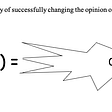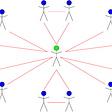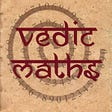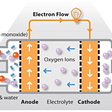# Absorbing Markov Chain: Limiting Matrix

I recently came across an interesting problem that required some understanding of Absorbing Markov Chains. The objective to calculate the percentages(in the long run) of ending states given an initial state. The input is a frequency table where each state has counts of transitions based on its index. To elaborate on the problem, I have created a visual explanation of the frequency table.

For this blog, I will break the solution into three parts while using Pandas to visualize the tables and NumPy for linear algebra. The goal is to return a limiting matrix, as shown above. For a more detailed explanation of the Absorbing Markov Chain, I highly recommend watching parts 7–9 of the Markov Chain series here.

1. Restructure
2. Normalize
3. Calculate FR

## Restructure

The indices of the frequency table indicate the observed state with the transition state. For example, table[1, 0] would return the count of transitions from state1 to state0(4). Some observed states have all 0 counts, indicating that the state is ‘terminal’; no longer changes state. Rows with all 0s equate to only one end-state to itself and therefore, I will populate the terminal states with 1, transitioning to itself.

After populating the terminal states, I will need to restructure, so the terminal states are on the table’s top-left. (Please note the shift in both the rows and columns.) Doing so will create two sub-matrices, R and Q, which will be needed to compute the limiting matrix at the end.

## Normalize

The inputs are in counts and need to be normalized, row-wise.

## Calculate FR

FR is the dot product of F and R, where F is the inverse of the (identity of Q) minus Q. More notably, it’s the bottom left portion that will complete the limiting matrix. The F, inverse calculation, can be solved with NumPy as such.

The values of the limiting matrix represent percentages of ending states(columns) given a starting state(index). For example, if the starting state was at 1, the end state probabilities would be:
2: 0%
3: 42.86%
4: 28.57%
5: 28.57%
0: 0%
1: 0%

--

--

--

## More from Albert Um

Hello! My name is Albert Um.

Love podcasts or audiobooks? Learn on the go with our new app.

## The Shortest Distance Between Two Points## Rule of Seventy## Shor’s Algorithm: The Enigma of a Brobdingnagian Computation## How to Convince A fanboy?## The Friendship Theorem## Vedic Math — The World’s Fastest Mental Math System## How to Avoid Math## Albert Um

Hello! My name is Albert Um.

## Why You Can’t Ditch The Car If You Live In A Rural Area## Clean Energy vs Fossil Fuels: A Revolutionary Discovery from MIT May Help Renewable Energy!## Bifurcation of hydrogen energy## Running for a better life